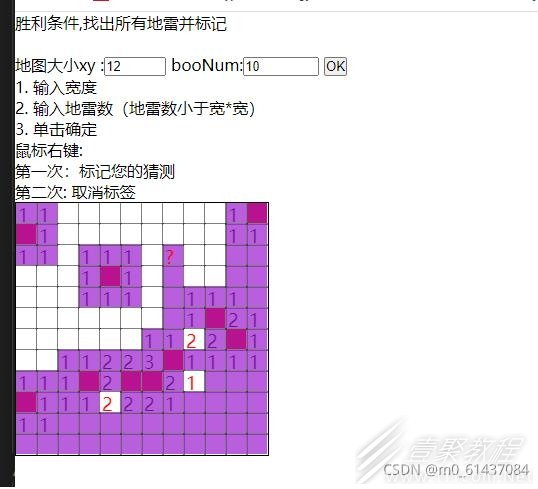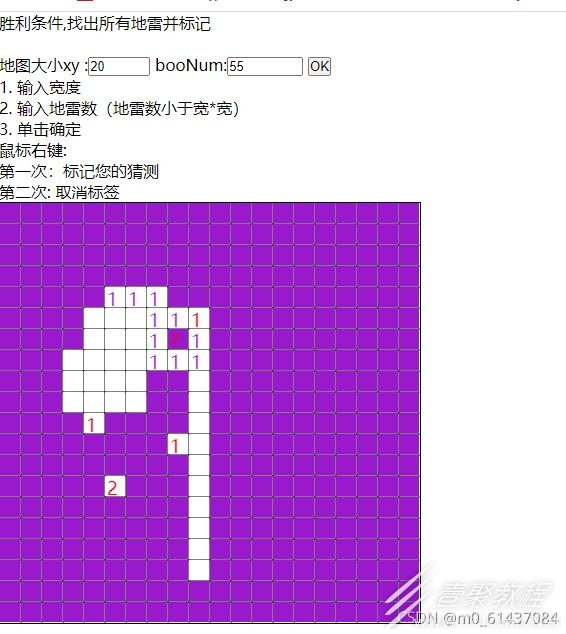# 利用js+canvas实现扫雷游戏代码示例

```

胜利条件,找出所有地雷并标记

地雷数量必须小于地图大小xy的平方

地图大小xy :
booNum:

1. 输入宽度 2. 输入地雷数（地雷数小于宽*宽）  3. 单击确定
鼠标右键:
第一次：标记您的猜测
第二次: 取消标签

let xy = document.getElementById('xyNum');
let boo = document.getElementById('booNum');
let meg = document.getElementById("message");
let div = document.getElementById('game');

//获取输入的宽高和地雷数
createContent = function (){
// console.log(xy.value);
// console.log(boo.value);
let xyNum = xy.value;
let booNum = boo.value;
// console.log(Math.pow(xyNum,2));

//判断输入是否合法
if(Math.pow(xyNum,2)<boo.value){
meg.style.display = 'block';
}
else {//绘制地图
div.innerHTML = '';//清除上次div里的地图
let game = new Game('game',xyNum,booNum);
}
}

```

lei.js

```/* 一个自定义原型数组方法  可以放到html里

arr:要找数组第一第二项 找到返回下标，没有返回-1
PS：只要this数组和arr数组第一第二项的值相等，即为找到。
*/
Array.prototype.myindexOf = function(arr){
for(let i=0;i{
if(this.booArrs.length ==this.markArrs.length){
for(let i=0;i{
return this.markArrs.myindexOf(this.booArrs[i])!=-1;
})){
this.booNum--;
}
if(this.booNum==0){
clearInterval(this.tim);
}
}
}
},10)

}
isboo(ctx,x,y,booArrs,numArrs,markArrs,xyNum){
new Isboo(ctx,x,y,booArrs,numArrs,markArrs,xyNum);
}

/*标记
*/
markObs(x,y){
console.log(x,y);
new MarkObs(this.ctx,x,y,this.booArrs,this.divw,this.markArrs);

}

}```

isboo.js

```Array.prototype.myindexOf = function(arr){
for(let i=0;i=0)&&(markArrs.myindexOf([x,y])==-1)){
ctx.clearRect(x*21,y*21,20,20);
x-=1;
// this.isbool(ctx,x,y,booArrs,numArrs,markArrs,xyNum);
this.isboor(ctx,x,y,booArrs,numArrs,markArrs,xyNum);
this.isboot(ctx,x,y,booArrs,numArrs,markArrs,xyNum);
this.isboob(ctx,x,y,booArrs,numArrs,markArrs,xyNum);
}else {
return ;
}
}
isboot(ctx,x,y,booArrs,numArrs,markArrs,xyNum){
if((numArrs.myindexOf([x,y])==-1)&&(y=0)&&(markArrs.myindexOf([x,y])==-1)){
ctx.clearRect(x*21,y*21,20,20);
y-=1;
// this.isbool(ctx,x,y,booArrs,numArrs,markArrs,xyNum);
// this.isboor(ctx,x,y,booArrs,numArrs,markArrs,xyNum);
// this.isboot(ctx,x,y,booArrs,numArrs,markArrs,xyNum);
this.isboob(ctx,x,y,booArrs,numArrs,markArrs,xyNum);
}else {
return ;
}
}

}```

MarkObs.js

```Array.prototype.myindexOf = function(arr){
for(let i=0;i

页面效果

初始化障碍物设置了透明度时正常游戏时

这里点击右键标记后忘了把填充颜色设置回来。所以后面变红。相关文章

《CF》工坊赏金令周任务更新时间
10-01

迷你世界2022年9月30日全新激活码免费领取
10-01

粉笔个性化服务怎么关
10-01

《原神》莱依拉命座效果一览
10-01

《召唤与合成2》新手策略-新手入门教程
10-01

无期迷途找不同攻略-无期迷途梦影藏瑕找不同怎么玩
10-01

```